Magnetic moments and Structure

Electromagnetic dipole moments are typically

µ ~ charge x length

For point particles, the intrinsic length is the Compton wavelength (i.e. 1/m)

µ ~ charge / mass = Q/m

Classically, the magnetic moment of a particle in a circular orbit with angular momentum L is

µ = (Q/2m) L

Subatomic particle magnetic moments are usually written

µ = g (Q/2m) J
• For a classical point particle: g = 1
• For structureless spin-1/2 point fermions: g = 2
• For structureless neutral particle: m = 0

 Particle gmeasured gpoint(QED theory) electron 2.002319304377(4) 2.002319304402(54) muon 2.0023318480(170) 2.0023318412(40) proton 5.58569478(12) 2.002 neutron m = 3.8260856(10) mN 0

Deviations from g=2 indicate that the fermion has structure. Even point fermions have a some structure due to the constant radiation and absorption of photons.

Protons and neutron do not have point particle dipole moments because they are not point particles. The magnetic moments of particles made from bound consituents are typically:

µ ~ Si gi(Qi/mi(eff))Ji

where the sum is over all the constuents. The effective mass of a constituent, mi(eff), depends on the intrinsic mass, mi, and on the binding forces on the constituents, i.e.

mi(eff) ~ mi(intrinsic) + mi(binding)

In an atom, the magnetic moments of paired (spin up - spin down) electrons cancel out, and the binding energy of any unpaired electron is typical 10 eV. In a nucleon, the the binding forces contribute several hundred MeV to the effective masses of the constituent quarks. If the constituents are not in an S-wave state, one would also expect contributions to the magnetic moment of order Qi·R, where R is the size of the particle.

Magnetic moments provide indirect but very powerful information about particle structure. Even in the undergraduate lab, measurements of Electron spin resonance and Nuclear magnetic resonance indicate that electrons are point particles and protons are not.

Scattering experiments are used to directly measure the size and substructure of particles.

Rutherford's Experiment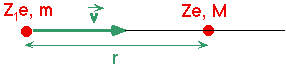Consider a light charged particle approaching a infinitely massive (i.e. fixed) charged particle.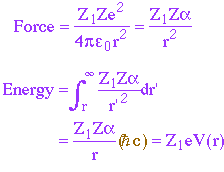The energy, U, needed for particle m to approach within a distance r of the target particle M is

U = (Z1Z/r)(1/137.03)(197 MeV fm)
= 1.44 (Z1Z/r) MeV fm

i.e. Umax Þ infinity as rÞ0 for point particles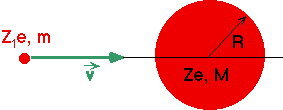If the target particle is a uniform charged sphere of radius R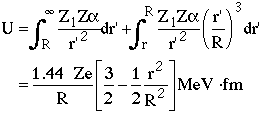\ Umax = (3/2) 1.44 Z1Z/R MeV fmRutherford thought: R~1Å (atom size), Z1=2 alpha particles, Z=79 gold foil.

Þ Umax = 3.4 KeV

but the alphas had kinetic energies ~5.5 MeV, so Rutherford observed backscattering

Þ R<10-3 Å

or

new billiard ball force

(Billiard balls, or any hard interaction, can scatter up to the kinematically allowed maximum).

In order to resolve this issue more data and more detailed analysis are necessary.

Classical Billiard Ball Scattering

An example of scattering via a strong, short range (contact) force (cf. Rutherford scattering).

Non-relativistic elastic scattering of a billiard ball (mass m, radius R) from a fixed (infinitely massive) billiard ball (radius R also).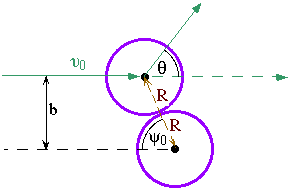By geometry, the angle y0 at the point of closest approach (i.e. the point of contact) is given by

sin(y0) =b/(2R)

and the scattering angle is

q = p-2y0

Consider a parallel beam of billiard balls with velocity v0 incident on a thin target which contains n target balls per unit volume and has a thickness L. Let N0 be the number of balls incident per unit area of the target. For a thin target, the number of particles incident with impact parameters between b & (b+|db|) will be dN= N0(2pb |db|)(nL); this is also the number of particles per unit area into an angular cone between q & (q +d q), so using

b = 2R sin(y0)

db = 2R cos(y0) dy0

sin(q) = 2 sin(y0) cos(y0)

dq = -2 dy0

we get

dN= N0[2p·2R sin(y0) 2R cos(y0) dq/2](nL)

\ dN= nLN0 2pR2 sin(q) dq

The scattering is independent of j, so it is uniform over j=0 to 2p, and so the number of particles scattered into a solid angle dW=dj sinq dq is

dN= nLN0 2pR2 sin(q) dq dj/(2p)

\ dN= nLN0 R2 dW

The flux of balls per unit time through unit area normal to the beam is

F= nball v

where nball is thedensity of balls in the beam. (N0=F dt, where dt is a time interval). The rate of scattering per unit target ball per unit time is thus

(dN/dt)/nL = F R2 dW

The scattering rate per unit target ball is

dW = F ds

where

ds/dW = R2

is known as the differential cross section. In general, the scattering or interaction rate for any process per target particle is

Rate = F s

i.e. s parameterizes the scattering probability and is known as the cross section. The total cross section is

s =(ds/dW) dW =R2dj sinq dq = 4pR2

as expected

The differential cross section can be also written in terms of the momentum transfer in the collison, q, i.e.

q = pf - p0

\ q2 = |q|2 = pf2 + p02 - 2 pf p0 cos q

For elastic scattering (pf=p0)

\ q2 = |q|2 = 2p02(1-cos q) = 4p02 sin2(q/2)

\ dq2 = 4p02 sin(q/2) cos(q/2) dq = 2p02 sin q dq

Since the cross section is independent of j, we can it integrate out (j=0 to 2p)

\ ds/dq2 = (p/p02) ds/dW

In this case the differential cross section is constant

ds/dq2 = pR2/p02

up to the maximum momentum transfer when a ball scatters straight back, i.e. pf = -p0 and q2max = 4p02

stot =pR2/p02 dq2 = 4pR2

once again as expected